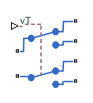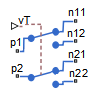# DPDT Switch

Double-pole double-throw switch

•Libraries:
Simscape / Electrical / Switches & Breakers

## Description

The DPDT Switch block models a double-pole double-throw switch:• When the switch is closed, ports p1 and p2 are connected to ports n12 and n22, respectively.

• When the switch is open, ports p1 and p2 are connected to ports n11 and n21, respectively.

Closed connections are modeled by a resistor with value equal to the Closed resistance parameter value. Open connections are modeled by a resistor with value equal to the reciprocal of the Open conductance parameter value.

If the Threshold width parameter is set to zero, the switch is closed if the voltage presented at the vT control port exceeds the value of the Threshold parameter.

If the Threshold width parameter is greater than zero, then switch conductance G varies smoothly between off-state and on-state values:

`$G=\frac{x}{{R}_{closed}}+\left(1-x\right){G}_{open}$`

The block uses the function 3λ2 – 2λ3 because its derivative is zero for λ = 0 and λ = 1.

Defining a small positive Threshold width can help solver convergence in some models, particularly if the control port signal vT varies continuously as a function of other network variables. However, defining a nonzero threshold width precludes the solver making use of switched linear optimizations. Therefore, if the rest of your network is switched linear, set Threshold width to zero.

Optionally, you can add a delay between the point at which the voltage at vT passes the threshold and the switch opening or closing. To enable the delay, on the Dynamics tab, set the Model dynamics parameter to ```Model turn-on and turn-off times```.

## Ports

Refer to the figure for port locations.### Input

expand all

Physical port associated with the signal that opens and closes the switch.

### Conserving

expand all

Electrical conserving port.

Electrical conserving port.

Electrical conserving port.

Electrical conserving port.

Electrical conserving port.

Electrical conserving port.

## Parameters

expand all

### Main

Resistance between the p and n electrical ports when the switch is closed.

Conductance between the p and n electrical ports when the switch is open. The value must be greater than zero.

The threshold voltage for the control physical signal input vT above which the switch will turn on.

The minimum increase in the control signal vT above the threshold value that will move the switch from fully open to fully closed.

### Dynamics

Select whether the block models a switching delay:

• `No dynamics` — Do not model the delay. This is the default option.

• ```Model turn-on and turn-off times``` — Use additional parameters to model a delay between the point at which the voltage at vT passes the threshold and the switch opening or closing.

Time between the input voltage exceeding the threshold voltage and the switch closing. The value must be greater than zero.

#### Dependencies

This parameter is visible only when you select ```Model turn-on and turn-off times``` for the Model dynamics parameter.

Time between the input voltage falling below the threshold voltage and the switch opening. The value must be greater than zero.

#### Dependencies

This parameter is visible only when you select ```Model turn-on and turn-off times``` for the Model dynamics parameter.

The value of the physical signal input vT at time zero. This value is used to initialize the delayed control voltage parameter internally.

#### Dependencies

This parameter is visible only when you select ```Model turn-on and turn-off times``` for the Model dynamics parameter.

## Version History

Introduced in R2012b